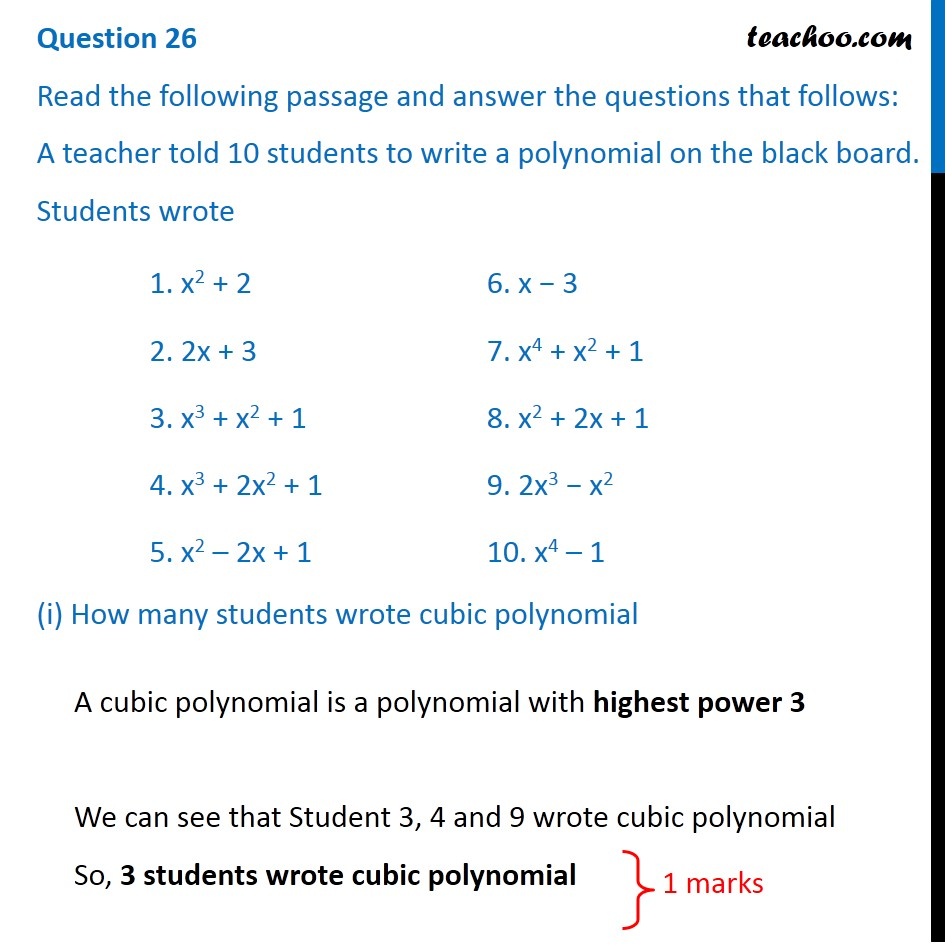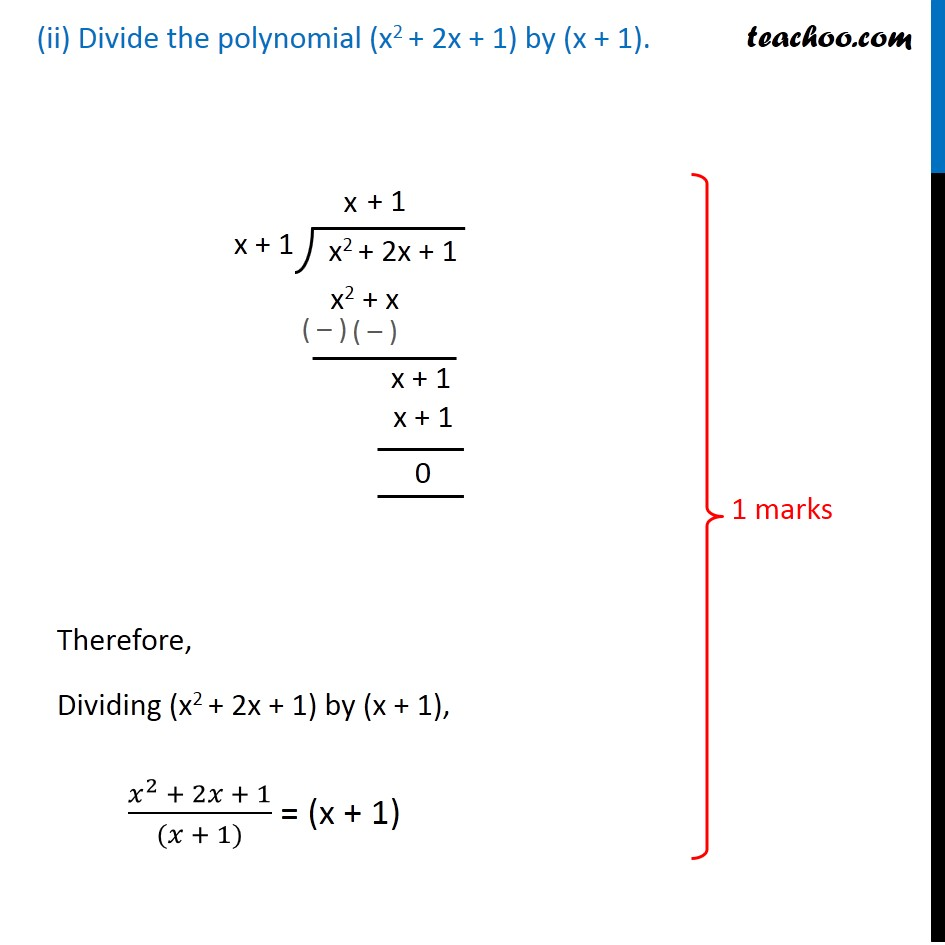CBSE Class 10 Sample Paper for 2020 Boards - Maths Basic

Class 10
Solutions of Sample Papers for Class 10 Boards

## (ii) Divide the polynomial (x 2 + 2x + 1) by (x + 1).Learn in your speed, with individual attention - Teachoo Maths 1-on-1 Class

### Transcript

Question 26 Read the following passage and answer the questions that follows: A teacher told 10 students to write a polynomial on the black board. Students wrote (i) How many students wrote cubic polynomial A cubic polynomial is a polynomial with highest power 3 We can see that Student 3, 4 and 9 wrote cubic polynomial So, 3 students wrote cubic polynomial (ii) Divide the polynomial (x2 + 2x + 1) by (x + 1). Therefore, Dividing (x2 + 2x + 1) by (x + 1), (𝑥^2 + 2𝑥 + 1)/((𝑥 + 1)) = (x + 1)